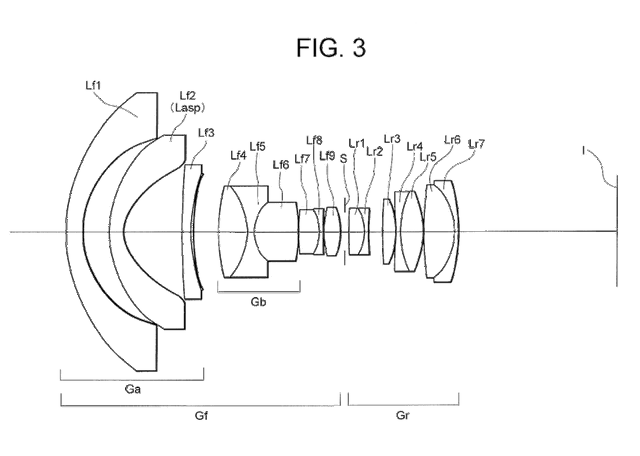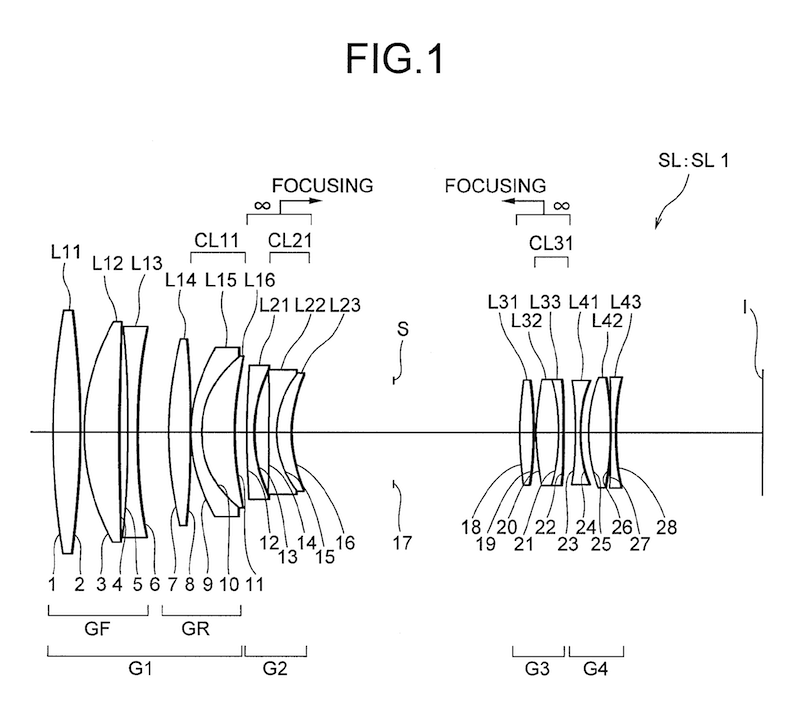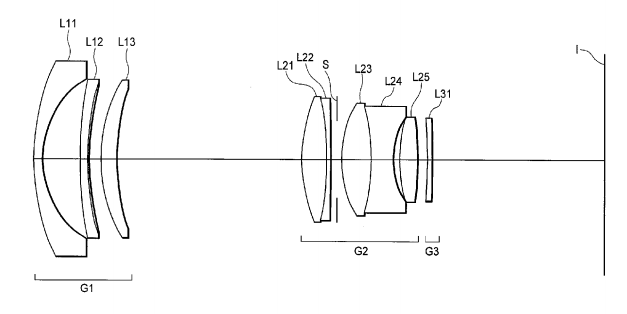# Interesting Nikon lens patents (incl. 10mm f/4 FX lens)

Three interesting lens patent applications were just published. The first one 20110109974 is for a 10mm f/4 full frame lens:• Example 1 is for a 17 mm f/4. Interestingly the image height is 33mm. This makes sense for a tilt-shift with +-12 mm of shift:

F0 = 17.11 FNO = 4.08 .omega. = 63.03.degree. Y = 33.00 TL = 188.30 BF = 53.036

• Examples 2 - 10 are for a 10 mm f/4 FX rectilinear lens. The images are from example 2. The field of view angle is an incredible 130 deg:

F0 = 10.3 FNO = 4.17 .omega. = 64.84.degree. Y = 21.6 TL = 132.78 BF = 38.10

Patent application 20110109979 is for a collection of full frame macro lenses. Note β, "where β (negative) denotes an available shooting magnification". All these have a maximum 1:1 (β = -1). This also explains why so many elements. The current 105 micro is a 14/12 design.• Example 1: f = 199.96976 FNO = 2.46, 15/12 design (f=focal length, FNO=aperture)
• Example 2: f = 169.98735 FNO = 2.50, 13/10 design
• Example 3: f = 198.00003 FNO = 3.21, 14/11 design
• Example 4: f = 219.98771 FNO = 2.04, 14/12 design
• Example 5: f = 160.01928 FNO = 2.87, 12/9 design

Patent application 20110109977 contains 10 different lens examples, most are for a 18-55mm f/3.5-5.6 DX, but examples 3 and 4 are for a FX ~28-90mm f/4-5.6, 10/7 design. Sounds like an FX kit lens, maybe for the D700 replacement or another low end full frame camera? Example 10 is a fixed 18mm f/3.5 DX lens.• Example 3: f = 29.0 50.0 91.7 FNO = 4.1 4.5 5.8 2.omega. 76.6 46.8 26.3 Y 21.6 21.6 21.6 TL 129.10 121.85 138.77 BF 38.91 51.29 75.04
• Example 10: f = 18.5 FNO = 3.7 2.omega. 78.0 Y 14.25 TL 131.37 Bf 38.35

Thanks Brian!

•